# Augmented Linear Model

#### 2021-09-21

ALM stands for “Augmented Linear Model.” The word “augmented” is used to reflect that the model introduces aspects that extend beyond the basic linear model. In some special cases alm() resembles the glm() function from stats package, but with a higher focus on forecasting rather than on hypothesis testing. You will not get p-values anywhere from the alm() function and won’t see $$R^2$$ in the outputs. The maximum what you can count on is having confidence intervals for the parameters or for the regression line. The other important difference from glm() is the availability of distributions that are not supported by glm() (for example, Folded Normal or Box-Cox Normal distributions) and it allows optimising non-standard parameters (e.g. $$\lambda$$ in Asymmetric Laplace distribution). Finally, alm() supports different loss functions via the loss parameter, so you can estimate parameters of your model via, for example, likelihood maximisation or via minimisation of MSE / MAE, using LASSO / RIDGE or by minimising a loss provided by user.

Although alm() supports various loss functions, the core of the function is the likelihood approach. By default the estimation of parameters in the model is done via the maximisation of likelihood function of a selected distribution. The calculation of the standard errors is done based on the calculation of hessian of the distribution. And in the centre of all of that are information criteria that can be used for the models comparison.

This vignette contains the following sections:

# Supported distributions

All the supported distributions have specific functions which form the following four groups for the distribution parameter in alm():

All of them rely on respective d- and p- functions in R. For example, Log Normal distribution uses dlnorm() function from stats package.

The alm() function also supports occurrence parameter, which allows modelling non-zero values and the occurrence of non-zeroes as two different models. The combination of any distribution from (1) - (3) for the non-zero values and a distribution from (4) for the occurrence will result in a mixture distribution model, e.g. a mixture of Log-Normal and Cumulative Logistic or a Hurdle Poisson (with Cumulative Normal for the occurrence part).

Every model produced using alm() can be represented as: $\begin{equation} \label{eq:basicALM} y_t = f(\mu_t, \epsilon_t) = f(x_t' B, \epsilon_t) , \end{equation}$ where $$y_t$$ is the value of the response variable, $$x_t$$ is the vector of exogenous variables, $$B$$ is the vector of the parameters, $$\mu_t$$ is the conditional mean (produced based on the exogenous variables and the parameters of the model), $$\epsilon_t$$ is the error term on the observation $$t$$ and $$f(\cdot)$$ is the distribution function that does a transformation of the inputs into the output. In case of a mixture distribution the model becomes slightly more complicated: $\begin{equation} \label{eq:basicALMMixture} \begin{matrix} y_t = o_t f(x_t' B, \epsilon_t) \\ o_t \sim \mathrm{Bernoulli}(p_t) \\ p_t = g(z_t' A) \end{matrix}, \end{equation}$ where $$o_t$$ is the binary variable, $$p_t$$ is the probability of occurrence, $$z_t$$ is the vector of exogenous variables and $$A$$ is the vector of parameters for the $$p_t$$.

In addition, the function supports scale model, i.e. the model that predicts the values of scale of distribution (for example, variance in case of normal distribution) based on the provided explanatory variables. This is discussed in some detail in a separate section.

The alm() function returns, along with the set of common for lm() variables (such as coefficient and fitted.values), the variable mu, which corresponds to the conditional mean used inside the distribution, and scale – the second parameter, which usually corresponds to standard error or dispersion parameter. The values of these two variables vary from distribution to distribution. Note, however, that the model variable returned by lm() function was renamed into data in alm(), and that alm() does not return terms and QR decomposition.

Given that the parameters of any model in alm() are estimated via likelihood, it can be assumed that they have asymptotically normal distribution, thus the confidence intervals for any model rely on the normality and are constructed based on the unbiased estimate of variance, extracted using sigma() function.

The covariance matrix of parameters almost in all the cases is calculated as an inverse of the hessian of respective distribution function. The exclusions are Normal, Log-Normal, Poisson, Cumulative Logistic and Cumulative Normal distributions, that use analytical solutions.

alm() function also supports factors in the explanatory variables, creating the set of dummies from them. In case of ordered variables (ordinal scale, is.ordered()), the ordering is removed and the set of dummies is produced. This is done in order to avoid the built in behaviour of R, which creates linear, squared, cubic etc levels for ordered variables, which makes the interpretation of the parameters difficult.

When the number of estimated parameters is calculated, in case of loss=="likelihood" the scale is considered as one of the parameters as well, which aligns with the idea of the maximum likelihood estimation. For all the other losses, the scale does not count (this aligns, for example, with how the number of parameters is calculated in OLS, which corresponds to loss="MSE").

Although the basic principles of estimation of models and predictions from them are the same for all the distributions, each of the distribution has its own features. So it makes sense to discuss them individually. We discuss the distributions in the four groups mentioned above.

## Density functions of continuous distributions

This group of functions includes:

For all the functions in this category resid() method returns $$e_t = y_t - \mu_t$$.

### Normal distribution

The density of normal distribution $$\mathcal{N}(\mu_t,\sigma)$$ is: $\begin{equation} \label{eq:Normal} f(y_t) = \frac{1}{\sqrt{2 \pi \sigma^2}} \exp \left( -\frac{\left(y_t - \mu_t \right)^2}{2 \sigma^2} \right) , \end{equation}$ where $$\sigma$$ is the standard deviation of the error term. This PDF has a very well-known bell shape: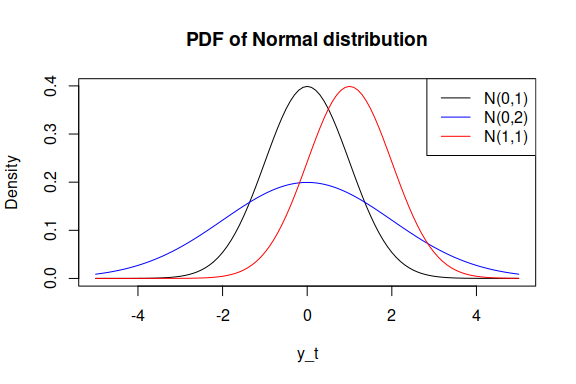alm() with Normal distribution (distribution="dnorm") is equivalent to lm() function from stats package and returns roughly the same estimates of parameters, so if you are concerned with the time of calculation, I would recommend reverting to lm().

Maximising the likelihood of the model is equivalent to the estimation of the basic linear regression using Least Squares method: $\begin{equation} \label{eq:linearModel} y_t = \mu_t + \epsilon_t = x_t' B + \epsilon_t, \end{equation}$ where $$\epsilon_t \sim \mathcal{N}(0, \sigma^2)$$.

The variance $$\sigma^2$$ is estimated in alm() based on likelihood: $\begin{equation} \label{eq:sigmaNormal} \hat{\sigma}^2 = \frac{1}{T} \sum_{t=1}^T \left(y_t - \mu_t \right)^2 , \end{equation}$ where $$T$$ is the sample size. Its square root (standard deviation) is used in the calculations of dnorm() function, and the value is then return via scale variable. This value does not have bias correction. However the sigma() method applied to the resulting model, returns the bias corrected version of standard deviation. And vcov(), confint(), summary() and predict() rely on the value extracted by sigma().

$$\mu_t$$ is returned as is in mu variable, and the fitted values are set equivalent to mu.

In order to produce confidence intervals for the mean (predict(model, newdata, interval="confidence")) the conditional variance of the model is calculated using: $\begin{equation} \label{eq:varianceNormalForCI} V({\mu_t}) = x_t V(B) x_t', \end{equation}$ where $$V(B)$$ is the covariance matrix of the parameters returned by the function vcov. This variance is then used for the construction of the confidence intervals of a necessary level $$\alpha$$ using the distribution of Student: $\begin{equation} \label{eq:intervalsNormal} y_t \in \left(\mu_t \pm \tau_{df,\frac{1+\alpha}{2}} \sqrt{V(\mu_t)} \right), \end{equation}$ where $$\tau_{df,\frac{1+\alpha}{2}}$$ is the upper $${\frac{1+\alpha}{2}}$$-th quantile of the Student’s distribution with $$df$$ degrees of freedom (e.g. with $$\alpha=0.95$$ it will be 0.975-th quantile, which, for example, for 100 degrees of freedom will be $$\approx 1.984$$).

Similarly for the prediction intervals (predict(model, newdata, interval="prediction")) the conditional variance of the $$y_t$$ is calculated: $\begin{equation} \label{eq:varianceNormalForPI} V(y_t) = V(\mu_t) + s^2 , \end{equation}$ where $$s^2$$ is the bias-corrected variance of the error term, calculated using: $\begin{equation} \label{eq:varianceNormalUnbiased} s^2 = \frac{1}{T-k} \sum_{t=1}^T \left(y_t - \mu_t \right)^2 , \end{equation}$ where $$k$$ is the number of estimated parameters (including the variance itself). This value is then used for the construction of the prediction intervals of a specify level, also using the distribution of Student, in a similar manner as with the confidence intervals.

### Laplace distribution

Laplace distribution has some similarities with the Normal one: $\begin{equation} \label{eq:Laplace} f(y_t) = \frac{1}{2 s} \exp \left( -\frac{\left| y_t - \mu_t \right|}{s} \right) , \end{equation}$ where $$s$$ is the scale parameter, which, when estimated using likelihood, is equal to the mean absolute error: $\begin{equation} \label{eq:bLaplace} \hat{s} = \frac{1}{T} \sum_{t=1}^T \left| y_t - \mu_t \right| . \end{equation}$ So maximising the likelihood is equivalent to estimating the linear regression via the minimisation of $$s$$ . So when estimating a model via minimising $$s$$, the assumption imposed on the error term is $$\epsilon_t \sim \mathcal{Laplace}(0, s)$$. The main difference of Laplace from Normal distribution is its fatter tails, the PDF has the following shape: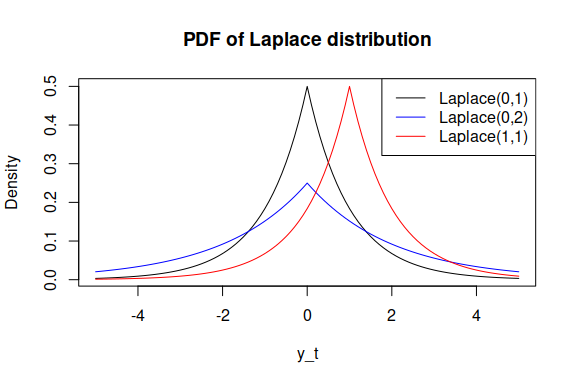alm() function with distribution="dlaplace" returns mu equal to $$\mu_t$$ and the fitted values equal to mu. $$s$$ is returned in the scale variable. The prediction intervals are derived from the quantiles of Laplace distribution after transforming the conditional variance into the conditional scale parameter $$s$$ using the connection between the two in Laplace distribution: $\begin{equation} \label{eq:bLaplaceAndSigma} s = \sqrt{\frac{\sigma^2}{2}}, \end{equation}$ where $$\sigma^2$$ is substituted either by the conditional variance of $$\mu_t$$ or $$y_t$$.

The kurtosis of Laplace distribution is 6, making it suitable for modelling rarely occurring events.

### Asymmetric Laplace distribution

Asymmetric Laplace distribution can be considered as a two Laplace distributions with different parameters $$s$$ for left and right side. There are several ways to summarise the probability density function, the one used in alm() relies on the asymmetry parameter $$\alpha$$ (Yu and Zhang 2005): $\begin{equation} \label{eq:ALaplace} f(y_t) = \frac{\alpha (1- \alpha)}{s} \exp \left( -\frac{y_t - \mu_t}{s} (\alpha - I(y_t \leq \mu_t)) \right) , \end{equation}$ where $$s$$ is the scale parameter, $$\alpha$$ is the skewness parameter and $$I(y_t \leq \mu_t)$$ is the indicator function, which is equal to one, when the condition is satisfied and to zero otherwise. The scale parameter $$s$$ estimated using likelihood is equal to the quantile loss: $\begin{equation} \label{eq:bALaplace} \hat{s} = \frac{1}{T} \sum_{t=1}^T \left(y_t - \mu_t \right)(\alpha - I(y_t \leq \mu_t)) . \end{equation}$ Thus maximising the likelihood is equivalent to estimating the linear regression via the minimisation of $$\alpha$$ quantile, making this equivalent to quantile regression. So quantile regression models assume indirectly that the error term is $$\epsilon_t \sim \mathcal{ALaplace}(0, s, \alpha)$$ (Geraci and Bottai 2007). The advantage of using alm() in this case is in having the full distribution, which allows to do all the fancy things you can do when you have likelihood.

Graphically, the PDF of asymmetric Laplace is: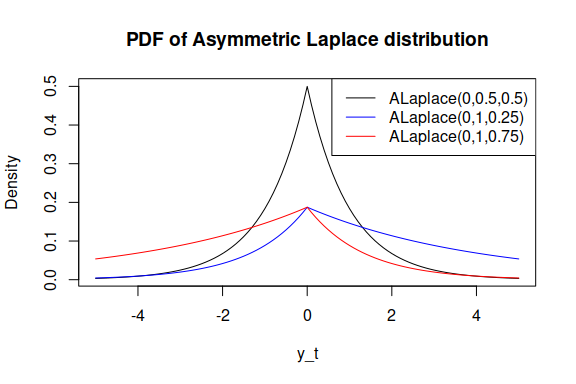In case of $$\alpha=0.5$$ the function reverts to the symmetric Laplace where $$s=\frac{1}{2}\text{MAE}$$.

alm() function with distribution="dalaplace" accepts an additional parameter alpha in ellipsis, which defines the quantile $$\alpha$$. If it is not provided, then the function will estimated it maximising the likelihood and return it as the first coefficient. alm() returns mu equal to $$\mu_t$$ and the fitted values equal to mu. $$s$$ is returned in the scale variable. The parameter $$\alpha$$ is returned in the variable other of the final model. The prediction intervals are produced using qalaplace() function. In order to find the values of $$s$$ for the holdout the following connection between the variance of the variable and the scale in Asymmetric Laplace distribution is used: $\begin{equation} \label{eq:bALaplaceAndSigma} s = \sqrt{\sigma^2 \frac{\alpha^2 (1-\alpha)^2}{(1-\alpha)^2 + \alpha^2}}, \end{equation}$ where $$\sigma^2$$ is substituted either by the conditional variance of $$\mu_t$$ or $$y_t$$.

NOTE: in order for the Asymmetric Laplace to work well, you might need to have large samples. This is inherited from the pinball score of the quantile regression. If you fit the model on 40 observations with $$\alpha=0.05$$, you will only have 2 observations below the line, which does not help very much with the fit. Similarly, the covariance matrix, produced via the Hessian might not be adequate in this situation (because there is not enough variability in the data due to extreme value of $$\alpha$$). The latter can be partially addressed by using bootstrap, but do not expect miracles on small samples.

### S distribution

The S distribution has the following density function: $\begin{equation} \label{eq:S} f(y_t) = \frac{1}{4 s^2} \exp \left( -\frac{\sqrt{|y_t - \mu_t|}}{s} \right) , \end{equation}$ where $$s$$ is the scale parameter. If estimated via maximum likelihood, the scale parameter is equal to: $\begin{equation} \label{eq:bS} \hat{s} = \frac{1}{2T} \sum_{t=1}^T \sqrt{\left| y_t - \mu_t \right|} , \end{equation}$ which corresponds to the minimisation of a half of “Mean Root Absolute Error” or “Half Absolute Moment.”

S distribution has a kurtosis of 25.2, which makes it a “severe excess” distribution (thus the name). It might be useful in cases of randomly occurring incidents and extreme values (Black Swans?). Here how the PDF looks: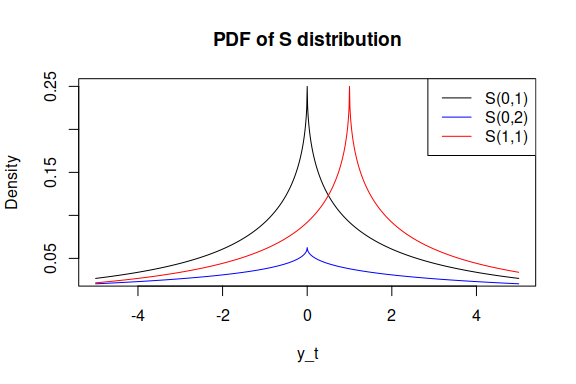alm() function with distribution="ds" returns $$\mu_t$$ in the same variables mu and fitted.values, and $$s$$ in the scale variable. Similarly to the previous functions, the prediction intervals are based on the qs() function from greybox package and use the connection between the scale and the variance: $\begin{equation} \label{eq:bSAndSigma} s = \left( \frac{\sigma^2}{120} \right) ^{\frac{1}{4}}, \end{equation}$ where once again $$\sigma^2$$ is substituted either by the conditional variance of $$\mu_t$$ or $$y_t$$.

### Generalised Normal distribution

The Generalised Normal distribution is a generalisation, which has Normal, Laplace and S as special cases. It has the following density function: $\begin{equation} \label{eq:gnormal} f(y_t) = \frac{\beta}{2s \Gamma(1/\beta)}\exp\left(-\left(\frac{|y_t - \mu|}{s}\right)^\beta\right), \end{equation}$ where $$s$$ is the scale and $$\beta$$ is the shape parameters. If estimated via maximum likelihood, the scale parameter is equal to: $\begin{equation} \label{eq:gnormalScale} \hat{s} = \sqrt[^\beta]{\frac{\beta}{T} \sum_{t=1}^T \left| y_t - \mu_t \right|^{\beta}} . \end{equation}$ In the special cases, this becomes either $$\sqrt{2}\times$$RMSE ($$\beta=2$$), or MAE ($$\beta=1$$) or a half of HAM ($$\beta=0.5$$). It is important to note that although in case of $$\beta=2$$, the distribution becomes equivalent to Normal, the scale of it will differ from the $$\sigma$$ (this follows directly from the formula above). The relations between the two is: $$s^2 = 2 \sigma^2$$.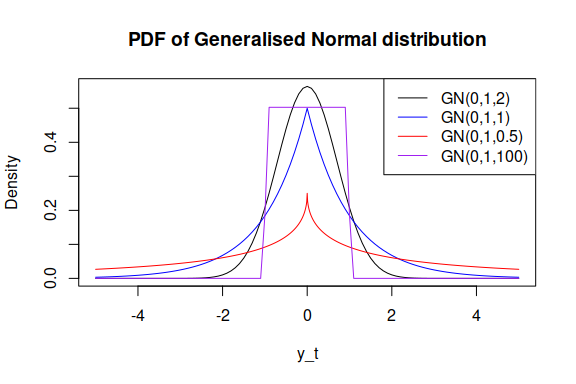The kurtosis of Generalised Normal distribution is determined by $$\beta$$ and is equal to $$\frac{\Gamma(5/\beta)\Gamma(1/\beta)}{\Gamma(3/\beta)^2}$$.

### Folded Normal distribution

Folded Normal distribution is obtained when the absolute value of normally distributed variable is taken: if $$x \sim \mathcal{N}(\mu, \sigma^2)$$, then $$|x| \sim \text{folded }\mathcal{N}(\mu, \sigma^2)$$. The density function is: $\begin{equation} \label{eq:foldedNormal} f(y_t) = \frac{1}{\sqrt{2 \pi \sigma^2}} \left( \exp \left( -\frac{\left(y_t - \mu_t \right)^2}{2 \sigma^2} \right) + \exp \left( -\frac{\left(y_t + \mu_t \right)^2}{2 \sigma^2} \right) \right), \end{equation}$ which can be graphically represented as: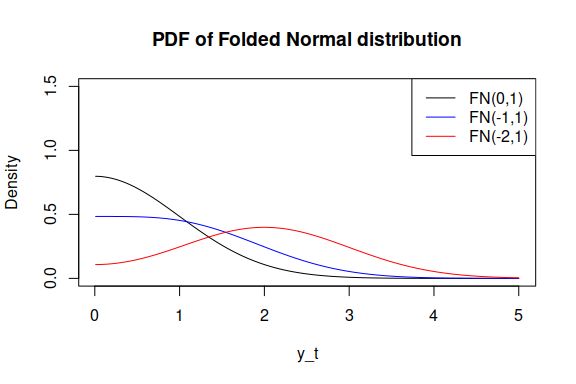Conditional mean and variance of Folded Normal are estimated in alm() (with distribution="dfnorm") similarly to how this is done for Normal distribution. They are returned in the variables mu and scale respectively. In order to produce the fitted value (which is returned in fitted.values), the following correction is done: $\begin{equation} \label{eq:foldedNormalFitted} \hat{y_t} = \sqrt{\frac{2}{\pi}} \sigma \exp \left( -\frac{\mu_t^2}{2 \sigma^2} \right) + \mu_t \left(1 - 2 \Phi \left(-\frac{\mu_t}{\sigma} \right) \right), \end{equation}$ where $$\Phi(\cdot)$$ is the CDF of Normal distribution.

The model that is assumed in the case of Folded Normal distribution can be summarised as: $\begin{equation} \label{eq:foldedNormalModel} y_t = \left| \mu_t + \epsilon_t \right|. \end{equation}$

The conditional variance of the forecasts is calculated based on the elements of vcov() (as in all the other functions), the predicted values are corrected in the same way as the fitted values , and the prediction intervals are generated from the qfnorm() function of greybox package. As for the residuals, resid() method returns $$e_t = y_t - \mu_t$$.

## Continuous distributions on a specific interval

There is currently only one distribution in this group:

### Logit-normal distribution

A random variable follows Logit-normal distribution if its logistic transform follows normal distribution: $\begin{equation} \label{eq:logitFunction} z = \mathrm{logit}(y) = \log \left(\frac{y}{1-y}) \right), \end{equation}$ where $$y\in (0,1)$$, $$y\sim \mathrm{logit}\mathcal{N}(\mu,\sigma^2)$$ and $$z\sim \mathcal{N}(\mu,\sigma^2)$$. The bounds are not supported, because the variable $$z$$ becomes infinite. The density function of $$y$$ is: $\begin{equation} \label{eq:logitNormal} f(y_t) = \frac{1}{\sqrt{2 \pi \sigma^2} y_t (1-y_t)} \exp \left( -\frac{\left(\mathrm{logit}(y_t) - \mu_t \right)^2}{2 \sigma^2} \right) , \end{equation}$ which has the following shapes: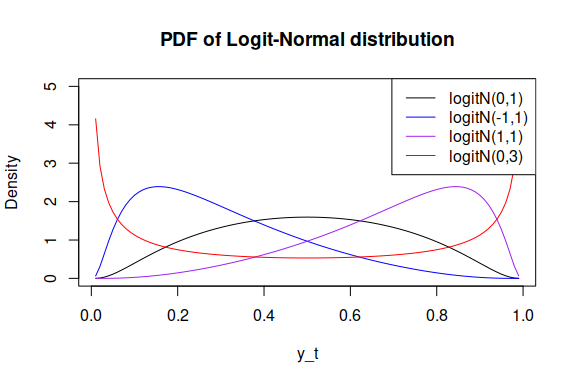Depending on the values of location and scale, the distribution can be either unimodal or bimodal and can be positively or negatively skewed. Because of its connection with normal distribution, the logit-normal has formulae for density, cumulative and quantile functions. However, the moment generation function does not have a closed form.

The scale of the distribution can be estimated via the maximisation of likelihood and has some similarities with the scale in Log Normal distribution: $\begin{equation} \label{eq:sigmaLogitNormal} \hat{\sigma}^2 = \frac{1}{T} \sum_{t=1}^T \left(\mathrm{logit}(y_t) - \mu_t \right)^2 . \end{equation}$

Estimating the model with Log Normal distribution is equivalent to estimating the parameters of logit-linear model: $\begin{equation} \label{eq:logitLinearModel} \mathrm{logit}(y_t) = \mu_t + \epsilon_t, \end{equation}$ where $$\epsilon_t \sim \mathcal{N}(0, \sigma^2)$$ or: $\begin{equation} \label{eq:logitLinearModelExp} y_t = \mathrm{logit}^{-1}(\mu_t + \epsilon_t), \end{equation}$ where $$\mathrm{logit}^{-1}(z)=\frac{\exp(z)}{1+\exp(z)}$$ is the inverse logistic transform.

alm() with distribution="dlogitnorm" does not transform the provided data and estimates the density directly using dlogitnorm() function from greybox package with the estimated mean $$\mu_t$$ and the variance . The $$\mu_t$$ is returned in the variable mu, the $$\sigma^2$$ is in the variable scale, while the fitted.values contains the inverse logistic transform of $$\mu_t$$, which, given the connection between the Normal and Logit-Normal distributions, corresponds to median of distribution rather than mean. Finally, resid() method returns $$e_t = \mathrm{logit}(y_t) - \mu_t$$.

### Beta distribution

Beta distribution is a distribution for a continuous variable that is defined on the interval of $$(0, 1)$$. Note that the bounds are not included here, because the probability density function is not well defined on them. If the provided data contains either zeroes or ones, the function will modify the values using: $\begin{equation} \label{eq:BetaWarning} y^\prime_t = y_t (1 - 2 \cdot 10^{-10}), \end{equation}$ and it will warn the user about this modification. This correction makes sure that there are no boundary values in the data, and it is quite artificial and needed for estimation purposes only.

The density function of Beta distribution has the form: $\begin{equation} \label{eq:Beta} f(y_t) = \frac{y_t^{\alpha_t-1}(1-y_t)^{\beta_t-1}}{B(\alpha_t, \beta_t)} , \end{equation}$ where $$\alpha_t$$ is the first shape parameter and $$\beta_t$$ is the second one. Note indices for the both shape parameters. This is what makes the alm() implementation of Beta distribution different from any other. We assume that both of them have underlying deterministic models, so that: $\begin{equation} \label{eq:BetaAt} \alpha_t = \exp(x_t' A) , \end{equation}$ and $\begin{equation} \label{eq:BetaBt} \beta_t = \exp(x_t' B), \end{equation}$ where $$A$$ and $$B$$ are the vectors of parameters for the respective shape variables. This allows the function to model any shapes depending on the values of exogenous variables. The conditional expectation of the model is calculated using: $\begin{equation} \label{eq:BetaExpectation} \hat{y}_t = \frac{\alpha_t}{\alpha_t + \beta_t} , \end{equation}$ while the conditional variance is: $\begin{equation} \label{eq:BetaVariance} \text{V}({y}_t) = \frac{\alpha_t \beta_t}{((\alpha_t + \beta_t)^2 (\alpha_t + \beta_t + 1))} . \end{equation}$ Beta distribution has shapes similar to the ones of Logit-Normal one, but with shape parameters regulating respectively the left and right tails of the distribution: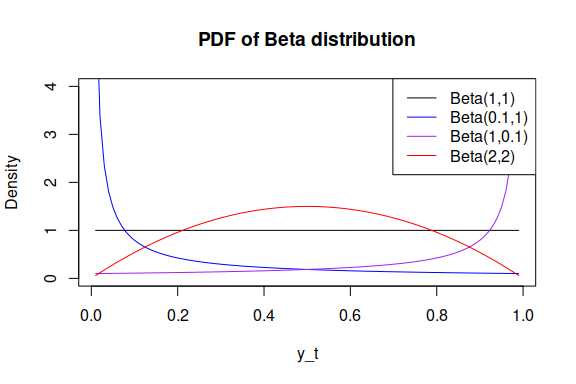alm() function with distribution="dbeta" returns $$\hat{y}_t$$ in the variables mu and fitted.values, and $$\text{V}({y}_t)$$ in the scale variable. The shape parameters are returned in the respective variables other$shape1 and other$shape2. You will notice that the output of the model contains twice more parameters than the number of variables in the model. This is because of the estimation of two models: $$\alpha_t$$ and $$\beta_t$$ - instead of one.

Respectively, when predict() function is used for the alm model with Beta distribution, the two models are used in order to produce predicted values for $$\alpha_t$$ and $$\beta_t$$. After that the conditional mean mu and conditional variance variances are produced using the formulae above. The prediction intervals are generated using qbeta function with the provided shape parameters for the holdout. As for the confidence intervals, they are produced assuming normality for the parameters of the model and using the estimate of the variance of the mean based on the variances (which is weird and probably wrong).

## Probability mass functions of discrete distributions

This group includes:

These distributions should be used in cases of count data.

### Poisson distribution

Poisson distribution used in ALM has the following standard probability mass function (PMF): $\begin{equation} \label{eq:Poisson} P(X=y_t) = \frac{\lambda_t^{y_t} \exp(-\lambda_t)}{y_t!}, \end{equation}$ where $$\lambda_t = \mu_t = \sigma^2_t = \exp(x_t' B)$$. As it can be noticed, here we assume that the variance of the model varies in time and depends on the values of the exogenous variables, which is a specific case of heteroscedasticity. The exponent of $$x_t' B$$ is needed in order to avoid the negative values in $$\lambda_t$$.

Here are several examples of the PMF of Poisson with different values of parameters $$\lambda$$: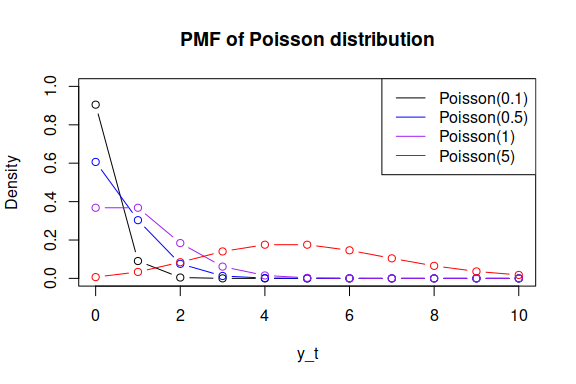alm() with distribution="dpois" returns mu, fitted.values and scale equal to $$\lambda_t$$. The quantiles of distribution in predict() method are generated using qpois() function from stats package. Finally, the returned residuals correspond to $$y_t - \mu_t$$, which is not really helpful or meaningful…

### Negative Binomial distribution

Negative Binomial distribution implemented in alm() is parameterised in terms of mean and variance: $\begin{equation} \label{eq:NegBin} P(X=y_t) = \binom{y_t+\frac{\mu_t^2}{\sigma^2-\mu_t}}{y_t} \left( \frac{\sigma^2 - \mu_t}{\sigma^2} \right)^{y_t} \left( \frac{\mu_t}{\sigma^2} \right)^\frac{\mu_t^2}{\sigma^2 - \mu_t}, \end{equation}$ where $$\mu_t = \exp(x_t' B)$$ and $$\sigma^2$$ is estimated separately in the optimisation process. These values are then used in the dnbinom() function in order to calculate the log-likelihood based on the distribution function.

Here are some examples of PMF of Negative Binomial distribution with different sizes and probabilities: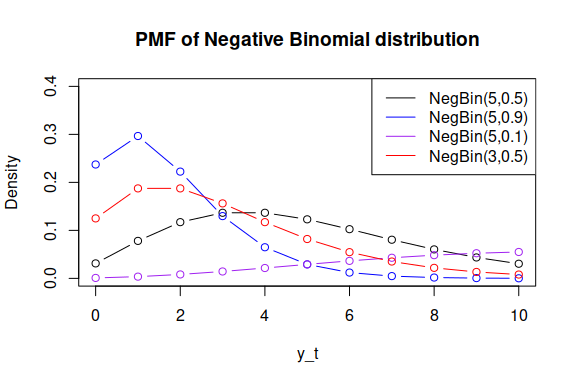alm() with distribution="dnbinom" returns $$\mu_t$$ in mu and fitted.values and $$\sigma^2$$ in scale. The prediction intervals are produces using qnbinom() function. Similarly to Poisson distribution, resid() method returns $$y_t - \mu_t$$. The user can also provide size parameter in ellipsis if it is reasonable to assume that it is known.

### An example of application

Round up the response variable for the next example:

xreg[,1] <- round(abs(xreg[,1]))
inSample <- xreg[1:180,]
outSample <- xreg[-c(1:180),]

Negative Binomial distribution:

ourModel <- alm(y~x1+x2, data=inSample, distribution="dnbinom")
summary(ourModel)
#> Response variable: y
#> Distribution used in the estimation: Negative Binomial with size=14.3158
#> Loss function used in estimation: likelihood
#> Coefficients:
#>             Estimate Std. Error Lower 2.5% Upper 97.5%
#> (Intercept)   6.2433     0.2078     5.8332      6.6535 *
#> x1            0.0040     0.0065    -0.0088      0.0168
#> x2           -0.0028     0.0039    -0.0104      0.0049
#>
#> Error standard deviation: 112.7588
#> Sample size: 180
#> Number of estimated parameters: 5
#> Number of degrees of freedom: 175
#> Information criteria:
#>      AIC     AICc      BIC     BICc
#> 2265.303 2265.648 2281.268 2282.163

And an example with predefined size:

ourModel <- alm(y~x1+x2, data=inSample, distribution="dnbinom", size=30)
summary(ourModel)
#> Response variable: y
#> Distribution used in the estimation: Negative Binomial with size=30
#> Loss function used in estimation: likelihood
#> Coefficients:
#>             Estimate Std. Error Lower 2.5% Upper 97.5%
#> (Intercept)   6.1903     0.1457     5.9028      6.4778 *
#> x1            0.0075     0.0045    -0.0015      0.0164
#> x2           -0.0024     0.0027    -0.0077      0.0030
#>
#> Error standard deviation: 112.4529
#> Sample size: 180
#> Number of estimated parameters: 4
#> Number of degrees of freedom: 176
#> Information criteria:
#>      AIC     AICc      BIC     BICc
#> 2330.133 2330.362 2342.905 2343.499

## Cumulative functions for binary variables

The final class of models includes two cases:

In both of them it is assumed that the response variable is binary and can be either zero or one. The main idea for this class of models is to use a transformation of the original data and link a continuous latent variable with the binary one. As a reminder, all the models eventually assume that: $\begin{equation} \label{eq:basicALMCumulative} \begin{matrix} o_t \sim \mathrm{Bernoulli}(p_t) \\ p_t = g(x_t' A) \end{matrix}, \end{equation}$ where $$o_t$$ is the binary response variable and $$g(\cdot)$$ is the cumulative distribution function. Given that we work with the probability of occurrence, the predict() method produces forecasts for the probability of occurrence rather than the binary variable itself. Finally, although many other cumulative distribution functions can be used for this transformation (e.g. plaplace() or plnorm()), the most popular ones are logistic and normal CDFs.

Given that the binary variable has Bernoulli distribution, its log-likelihood is: $\begin{equation} \label{eq:BernoulliLikelihood} \ell(p_t | o_t) = \sum_{o_t=1} \log p_t + \sum_{o_t=0} \log(1 - p_t), \end{equation}$ So the estimation of parameters for all the CDFs can be done maximising this likelihood.

In all the functions it is assumed that the probability $$p_t$$ corresponds to some sort of unobservable level’ $$q_t = x_t' A$$, and that there is no randomness in this level. So the aim of all the functions is to estimate correctly this level and then get an estimate of probability based on it.

The error of the model is calculated using the observed occurrence variable and the estimated probability $$\hat{p}_t$$. In a way, in this calculation we assume that $$o_t=1$$ happens mainly when the respective estimated probability $$\hat{p}_t$$ is very close to one. So, the error can be calculated as: $\begin{equation} \label{eq:BinaryError} u_t' = o_t - \hat{p}_t . \end{equation}$ However this error is not useful and should be somehow transformed into the original scale of $$q_t$$. Given that both $$o_t \in (0, 1)$$ and $$\hat{p}_t \in (0, 1)$$, the error will lie in $$(-1, 1)$$. We therefore standardise it so that it lies in the region of $$(0, 1)$$: $\begin{equation} \label{eq:BinaryErrorBounded} u_t = \frac{u_t' + 1}{2} = \frac{o_t - \hat{p}_t + 1}{2}. \end{equation}$

This transformation means that, when $$o_t=\hat{p}_t$$, then the error $$u_t=0.5$$, when $$o_t=1$$ and $$\hat{p}_t=0$$ then $$u_t=1$$ and finally, in the opposite case of $$o_t=0$$ and $$\hat{p}_t=1$$, $$u_t=0$$. After that this error is transformed using either Logistic or Normal quantile generation function into the scale of $$q_t$$, making sure that the case of $$u_t=0.5$$ corresponds to zero, the $$u_t>0.5$$ corresponds to the positive and $$u_t<0.5$$ corresponds to the negative errors. The distribution of the error term is unknown, but it is in general bimodal.

### Cumulative Logistic distribution

We have previously discussed the density function of logistic distribution. The standardised cumulative distribution function used in alm() is: $\begin{equation} \label{eq:LogisticCDFALM} \hat{p}_t = \frac{1}{1+\exp(-\hat{q}_t)}, \end{equation}$ where $$\hat{q}_t = x_t' A$$ is the conditional mean of the level, underlying the probability. This value is then used in the likelihood in order to estimate the parameters of the model. The error term of the model is calculated using the formula: $\begin{equation} \label{eq:LogisticError} e_t = \log \left( \frac{u_t}{1 - u_t} \right) = \log \left( \frac{1 + o_t (1 + \exp(\hat{q}_t))}{1 + \exp(\hat{q}_t) (2 - o_t) - o_t} \right). \end{equation}$ This way the error varies from $$-\infty$$ to $$\infty$$ and is equal to zero, when $$u_t=0.5$$.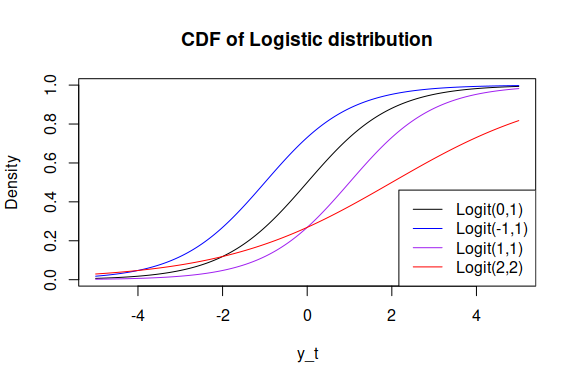The alm() function with distribution="plogis" returns $$q_t$$ in mu, standard deviation, calculated using the respective errors in scale and the probability $$\hat{p}_t$$ based on in fitted.values. resid() method returns the errors discussed above. predict() method produces point forecasts and the intervals for the probability of occurrence. The intervals use the assumption of normality of the error term, generating respective quantiles (based on the estimated $$q_t$$ and variance of the error) and then transforming them into the scale of probability using Logistic CDF. This method for intervals calculation is approximate and should not be considered as a final solution!

### Cumulative Normal distribution

The case of cumulative Normal distribution is quite similar to the cumulative Logistic one. The transformation is done using the standard normal CDF: $\begin{equation} \label{eq:NormalCDFALM} \hat{p}_t = \Phi(q_t) = \frac{1}{\sqrt{2 \pi}} \int_{-\infty}^{q_t} \exp \left(-\frac{1}{2}x^2 \right) dx , \end{equation}$ where $$q_t = x_t' A$$. Similarly to the Logistic CDF, the estimated probability is used in the likelihood in order to estimate the parameters of the model. The error term is calculated using the standardised quantile function of Normal distribution: $\begin{equation} \label{eq:NormalError} e_t = \Phi \left(\frac{o_t - \hat{p}_t + 1}{2}\right)^{-1} . \end{equation}$ It acts similar to the error from the Logistic distribution, but is based on the different set of functions. Its CDF has similar shapes to the logit: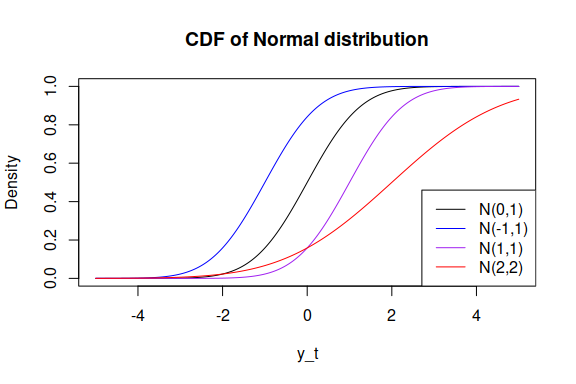Similar to the Logistic CDF, the alm() function with distribution="pnorm" returns $$q_t$$ in mu, standard deviation, calculated based on the errors in scale and the probability $$\hat{p}_t$$ based on in fitted.values. resid() method returns the errors discussed above. predict() method produces point forecasts and the intervals for the probability of occurrence. The intervals are also approximate and use the same principle as in Logistic CDF.

## Mixture distribution models

Finally, mixture distribution models can be used in alm() by defining distribution and occurrence parameters. Currently only plogis() and pnorm() are supported for the occurrence variable, but all the other distributions discussed above can be used for the modelling of the non-zero values. If occurrence="plogis" or occurrence="pnorm", then alm() is fit two times: first on the non-zero data only (defining the subset) and second - using the same data, substituting the response variable by the binary occurrence variable and specifying distribution=occurrence. As an alternative option, occurrence alm() model can be estimated separately and then provided as a variable in occurrence.

As an example of mixture model, let’s generate some data:

xreg[,1] <- round(exp(xreg[,1]-400) / (1 + exp(xreg[,1]-400)),0) * xreg[,1]
# Sometimes the generated data contains huge values
xreg[is.nan(xreg[,1]),1] <- 0;
inSample <- xreg[1:180,]
outSample <- xreg[-c(1:180),]

First, we estimate the occurrence model (it will complain that the response variable is not binary, but it will work):

modelOccurrence <- alm(y~x1+x2+Noise, inSample, distribution="plogis")

And then use it for the mixture model:

modelMixture <- alm(y~x1+x2+Noise, inSample, distribution="dlnorm", occurrence=modelOccurrence)

The occurrence model will be return in the respective variable:

summary(modelMixture)
#> Response variable: y
#> Distribution used in the estimation: Mixture of Log Normal and Cumulative logistic
#> Loss function used in estimation: likelihood
#> Coefficients:
#>             Estimate Std. Error Lower 2.5% Upper 97.5%
#> (Intercept)   5.2748     0.3302     4.6231      5.9265 *
#> x1            0.0025     0.0032    -0.0039      0.0088
#> x2            0.0006     0.0019    -0.0033      0.0044
#> Noise         0.0029     0.0010     0.0008      0.0049 *
#>
#> Error standard deviation: 0.1365
#> Sample size: 180
#> Number of estimated parameters: 5
#> Number of degrees of freedom: 175
#> Information criteria:
#>      AIC     AICc      BIC     BICc
#> 1883.561 1873.906 1915.490 1890.421
summary(modelMixture\$occurrence)
#> Response variable: y
#> Distribution used in the estimation: Cumulative logistic
#> Loss function used in estimation: likelihood
#> Coefficients:
#>             Estimate Std. Error Lower 2.5% Upper 97.5%
#> (Intercept)  -7.6510     7.8621   -23.1677      7.8658
#> x1            0.1331     0.0723    -0.0096      0.2758
#> x2            0.1504     0.0462     0.0593      0.2415 *
#> Noise         0.0034     0.0253    -0.0466      0.0534
#>
#> Error standard deviation: 1.144
#> Sample size: 180
#> Number of estimated parameters: 5
#> Number of degrees of freedom: 175
#> Information criteria:
#>      AIC     AICc      BIC     BICc
#> 167.6948 168.0396 183.6595 184.5549

We can also do regression diagnostics using plots:

par(mfcol=c(3,3))
plot(modelMixture, c(1:9))`